# Team:Evry/LogisticFunctions

Iron coli project

# Logistic functions

When we started to model biological behaviors, we realised very soon that we were going to need a function that simulates a non-exponential evolution, that would include a simple speed control and a maximum value. A smooth step function.

Such functions, named logistic functions were introduced around 1840 by M. Verhulst.These functions looked perfect, but we needed more control : we needed to set a starting value and a precision.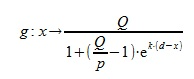## Parameters:

• Q : Magnitude.
The limit of g as x approaches infinity is Q.
• d : Threshold.
The value of x from which we consider the start of the phenomenon.
• p : Precision.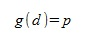Since the function never reaches 0 nor Q, we have to set an approximation for 0 or Q.
• k : Efficiency.
This parameter influences the length of the phenomenon.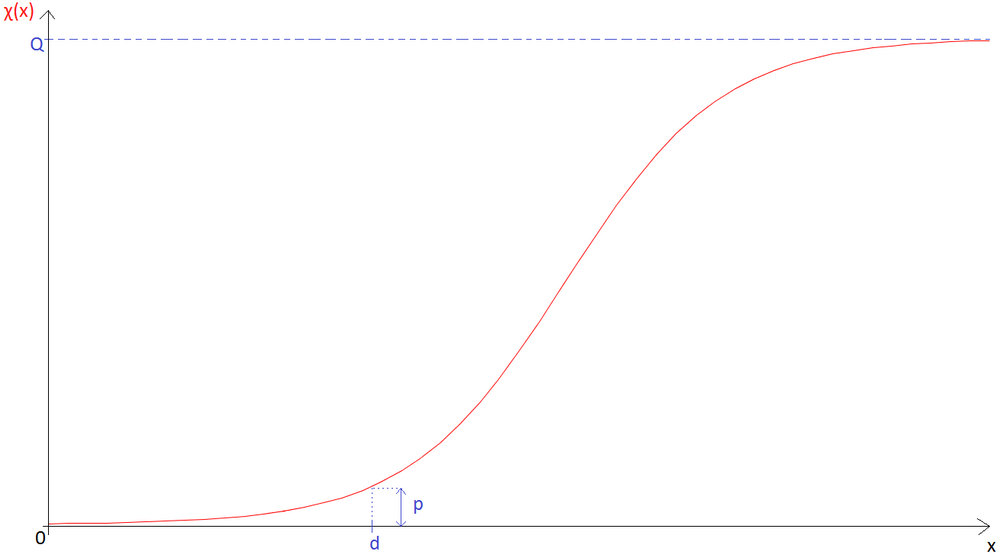## Differential form:

Let the following be a Cauchy problem: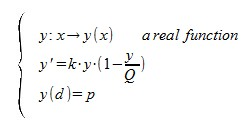The solution of this Cauchy problem is as below: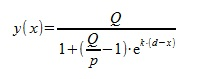Here is our logistic function. Yet, differential equations are not always time-related.
Let x be a temporal function, and y be a x-related logistic function. In order to integrate y into a temporal ODE, we need to write it differently: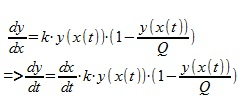But this equation can't be integrated in a temporal system like other equations. Because y depend on x. In our model, x is a state variable of the system. To implement this equation, we solve it before the entire system.